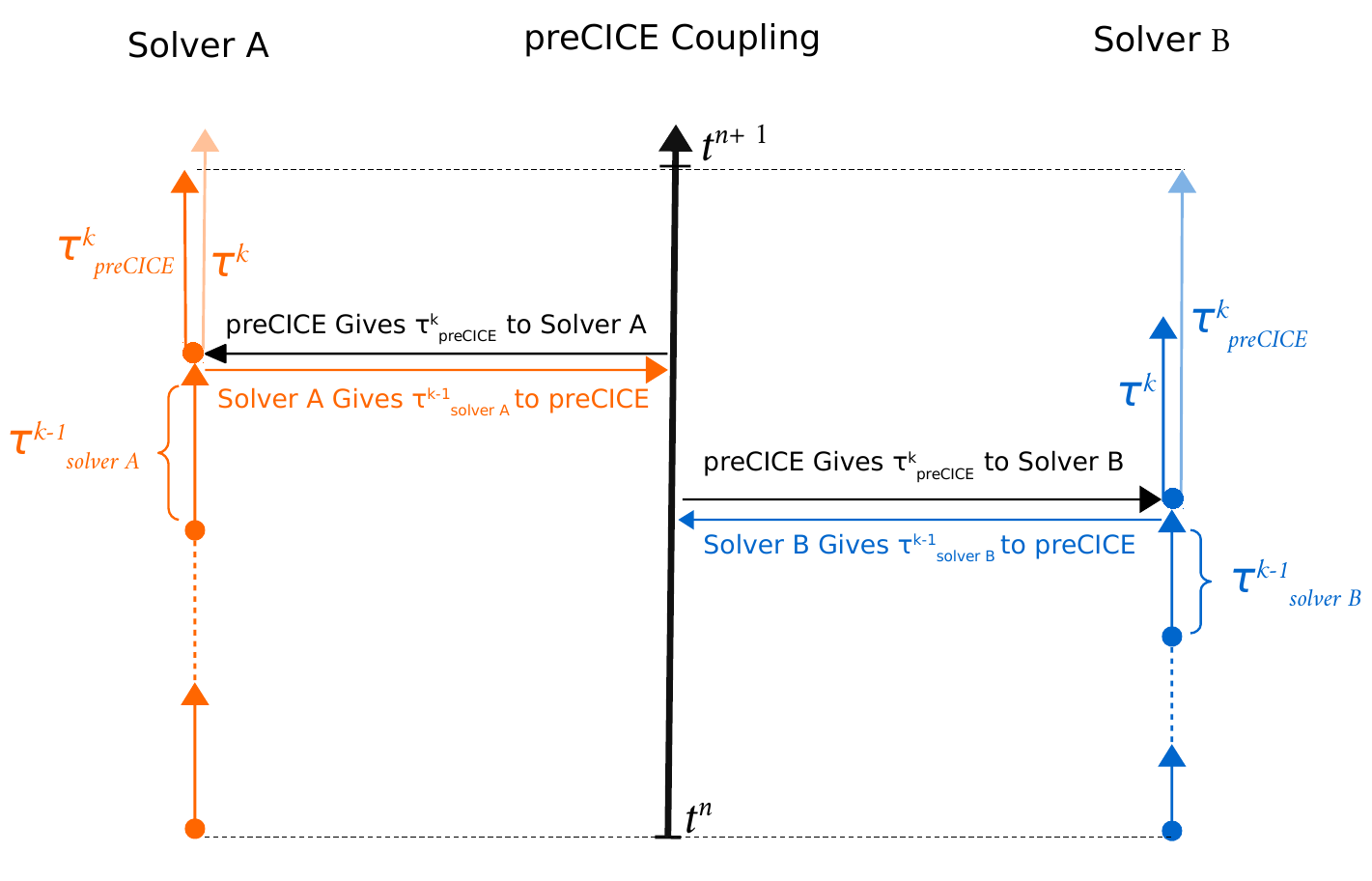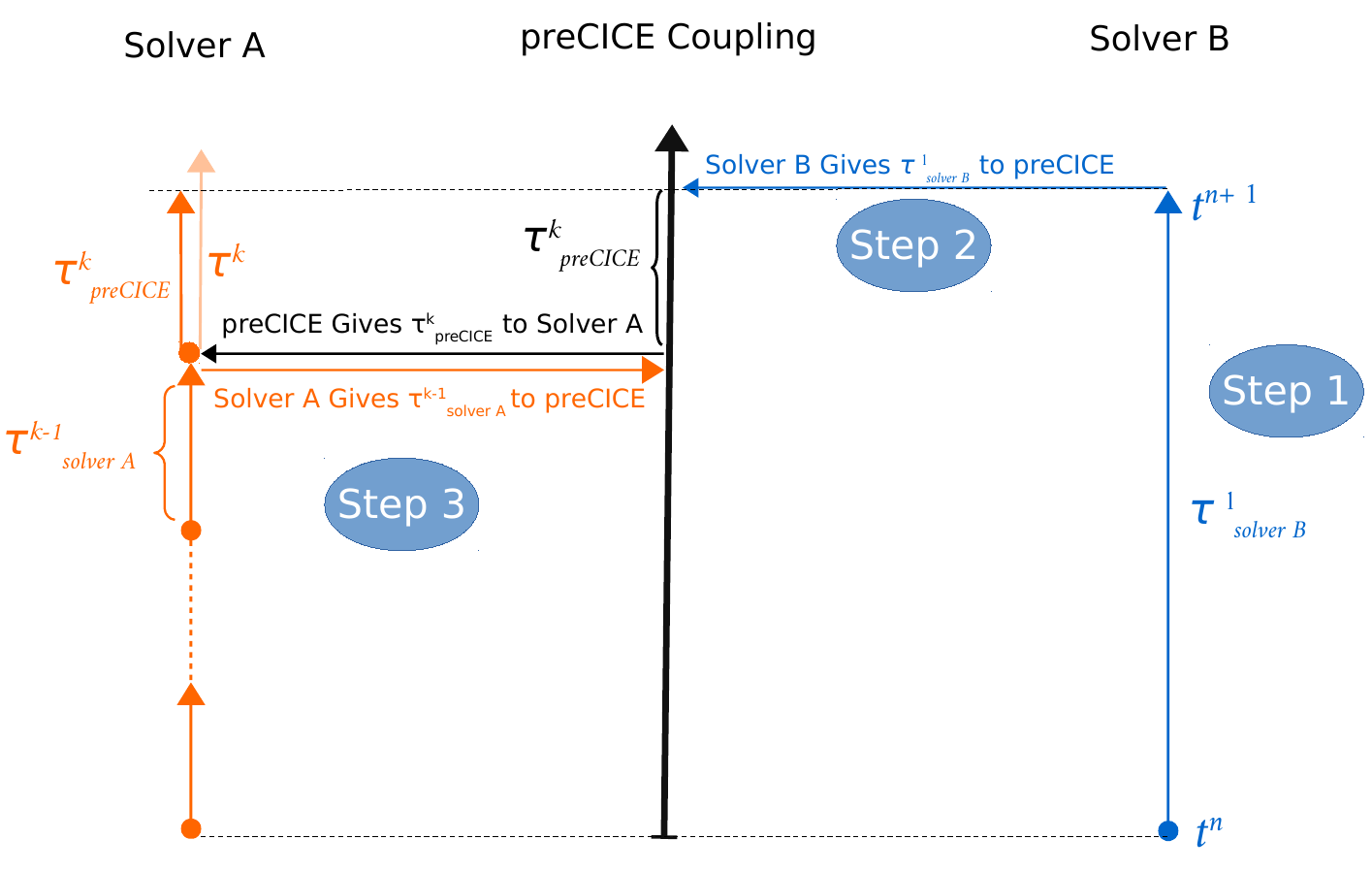In this step, you learn how preCICE handles non-matching time step sizes and a few more things about simulation time.

In previous steps, you have already seen that there are quite some things going on with time step sizes. Let us now have a look at what is actually happening.

...
double solverDt; // solver time step size
double preciceDt; // maximum precice time step size
double dt; // actual time step size

preciceDt = precice.initialize();
while (not simulationDone()){ // time loop
solverDt = beginTimeStep(); // e.g. compute adaptive dt
dt = min(preciceDt, solverDt);
solveTimeStep(dt);
endTimeStep(); // e.g. update variables, increment time
}


Good thing to know: in this step, you do really not have to alter your code. Everything needed is already there and can be configured. :relieved:

There are basically two options to choose from in the configuration.

<time-window-size value="..." method="..."/>


method can be:

• fixed: A fixed time window with size value is prescribed, during which both participants can use whatever time step sizes they want. Communication of coupling data happens only after each time window.
• first-participant: The first participant prescribes the time step size for the second one. Communication of coupling data happens after each time step. This option is only available for serial coupling schemes (more about configuration of coupling schemes). The attribute value is not applicable.

Let us have a closer look at both options.

## Fixed time window

The preCICE configuration defines a fixed time window. Both participants can use smaller time step sizes, but then they subcycle, i.e. coupling data is only communicated at the end of each time window. The figure below illustrates this procedure (k is the subcycling index, the dashed lines mark the time window):• After each time step, both participants tell preCICE which time step size dt they just used. This way, preCICE can keep track of the total time. preCICE returns the remainder time to the next window.
preciceDt = precice.advance(dt);

• Both participants compute their next (adaptive) time step size. It can be larger or smaller than the remainder preciceDt.
solverDt = beginTimeStep();


If it is larger, the remainder preciceDt is used instead (orange participant, dark orange is used). If it is smaller, the participant’s time step size solverDt can be used (blue participant, dark blue is used). These two cases are reflected in:

dt = min(preciceDt, solverDt)

• Once both participants reach the end of the time window, coupling data is exchanged.

If a participant subcycles it is actually not necessary to write data to or read data from preCICE. To avoid unnecessary calls, preCICE offers two optional helper functions:

bool isReadDataAvailable () const;
bool isWriteDataRequired (double computedTimeStepLength) const;


You can use them as follows:

while (not simulationDone()){ // time loop
solverDt = beginTimeStep(); // e.g. compute adaptive dt
dt = min(preciceDt, solverDt);
setDisplacements(displacements);
}
solveTimeStep(dt);
if (precice.isWriteDataRequired(dt)){
computeForces(forces);
precice.writeBlockVectorData(forceID, vertexSize, vertexIDs, forces);
}
endTimeStep(); // e.g. update variables, increment time
}


## First participant prescribes time step size

The first participant sets the time step size. This requires that the second participant runs after the first one. Thus, as stated above, this option is only applicable for serial coupling.• The blue participant B is first and computes a step with its time step size (Step 1).
• In advance, this time step size is given to preCICE (Step 2).
preciceDt = precice.advance(dt);

• preCICE has tracked the time level of the orange participant A and returns the remainder to reach B’s time step size.
• A computes its next (adaptive) time step size. It can now be larger or smaller than the remainder.
solverDt = beginTimeStep();


If it is larger, the remainder preciceDt is used instead (the case below in Step 3, dark orange is used). If it is smaller, the participant’s time step size solverDt can be used (not visualized). These two cases are again reflected in the formula:

dt = min(preciceDt, solverDt)

• The procedure starts over with the blue participant B.

## Steering the end of the simulation

One last thing about time. There is also a helper function in preCICE that allows you to steer the end of a coupled simulation:

bool isCouplingOngoing();


This functions looks at max-time-windows and max-time as defined in the preCICE configuration and knows when it is time to go. Then, you should call finalize. It replaces your simulationDone().

while (not precice.isCouplingOngoing()){ // time loop
...
}
precice.finalize();